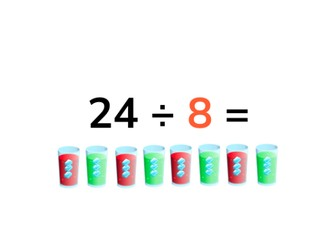Solving the division table of 8

# Solving the division table of 8

Students learn to solve the division table of 8.8,000 schools use Gynzy92,000 teachers use Gynzy1,600,000 students use Gynzy

## General

The students learn to solve the division table of 8.

## Standards

CCSS.Math.Content.3.OA.B.5/6

## Learning objective

Students will be able to solve the division table of 8.

## Introduction

You ask students to volunteer to fill in the jumps of 8 that the frog takes on the sand piles. Next you practice the division table of 8 with the students on the whiteboard. You do this forwards and backwards.

## Instruction

First you discuss the importance of calculating division problems. Next you explain that in a division problem you have a certain amount of something in each group and that with the division table of 8 you divide by 8 each time. For this you show an example of 16 ice cubes, that you are going to divide evenly between 8 glasses. From here you can use the bar on the bottom right to navigate through the lesson (visual, abstract, story) to discuss the lesson in the way that best suits your learning goals. Otherwise you may continue following the pages in order and start by discussing the problems with visual support. Here you explain how you solve a division problem by telling how a division problem works with images of ice cubes that must be divided between 8 glasses; next you practice by dividing a number of lady bugs between 8 leaves. After this you show images and the students must come up with the problem that matches each image. Then you show an image and ask a student volunteer to divide the monkeys between the trees and the pencils between the 8 containers. After this you have the students solve division problems on their own through an image. Next you discuss the abstract problems. First you show what a division problem with 8 looks like when you divide up the number 8 evenly. Then you show division problems with 8. Next the students practice using what they've learned. After this you walk the students through the steps of solving a story problem. The students can click on the speaker to hear the story.

Check whether the students can solve division problems from the division table of 8 by asking them the following questions:
- What does the problem 16 ÷ 8 mean?
- Can you tell an example of a division problem?

## Quiz

The students test their understanding of the division table of 8 through ten exercises. For some of the exercises there is visual support provided, some are abstract division problems and other exercises are story problems from the division table of 8.

## Closing

You have the students solve yet another problem with visual support, then an abstract problem and then they solve one more story problem. Finally you can play a ball game. A student names a problem from the division table of 8 and throws the ball to another student. The student who catches the ball says the answer and then another problem from the division table of 8, then passes the ball along to another student.

## Teaching tips

You can have the students solve division problems with concrete materials by having them divide them into 8 groups.

## Instruction materials

MAB blocks or other concrete materials for each pair of students.
A ball.

### The online teaching platform for interactive whiteboards and displays in schools

• Save time building lessons

• Manage the classroom more efficiently

• Increase student engagement